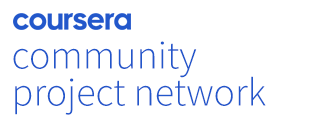# Basic calculations with Python in Kaggle

4.2
stars
21 ratings
Offered ByIn this Guided Project, you will:

Learn basic python syntax, variable assignment and numbers.

Define simple functions.

Perform simple mathematical calculations.

2 hrs
Beginner
Split-screen video
English
Desktop only

Basic calculations with Python in Kaggle : Python is the most widely used data science programming language. It is a cross-platform programming language, which means that it can run on multiple platforms like Windows, macOS, Linux. This general-purpose and dynamic language is inherently object-oriented. In this project, we will explore the basics and learn to perform mathematical calculations in Kaggle. Kaggle is the world's largest data science community with powerful tools and resources that helps in achieving the manifold data science goals. By the end of this project, you will learn basic python syntax, variable assignment and numbers, define simple functions and perform simple mathematical calculations. This Guided Project was created by a Coursera community member.

## Skills you will develop

Computer ProgrammingData SciencePython ProgrammingCalculations

## Learn step-by-step

In a video that plays in a split-screen with your work area, your instructor will walk you through these steps:

2. Sample Code and output

3. Basic python syntax, variable assignment and numbers

4. Simple functions and solving simple mathematics

5. Arithmetic operators and calculations

6. Booleans & Conditionals

7. Getting help in python

## How Guided Projects work